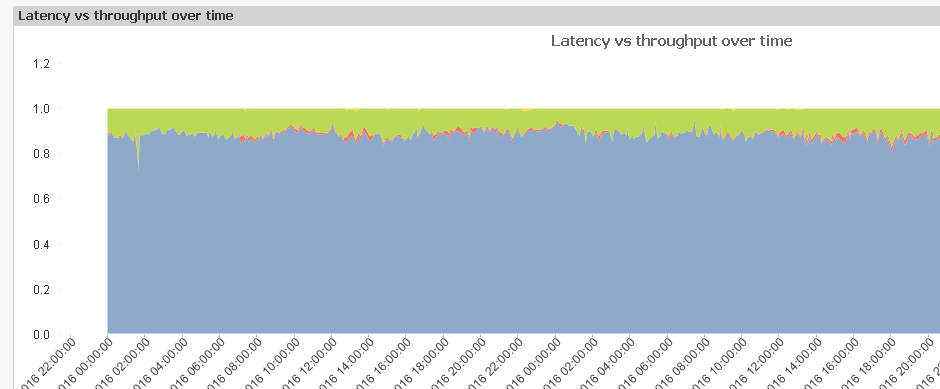# New to QlikView

Discussion board where members can get started with QlikView.

Announcements
Talk to Experts Tuesday, Live Q&A, September 22: Moving from QlikView to Qlik Sense. REGISTER
cancel
Showing results for
Did you mean:
HighlightedCreator

## showing percent in Y- axis

I have requirement to show the Y axis of line chart in percent, similar to Mekko Chart.

With some simple data I simply used count(data)/count(total(data) for the expression but  when I used it in a different larger sample with the following expression :

Count({<Latency = {'<600'}, [Throughput (Kbps)] = {'<1000'}>}DISTINCT [Hardware Id])/Count(DISTINCT([Hardware Id]))

and changed the number to integer and selected show in percent under number tab, the total number does not result in 100%.  In this example it shows below 100%, and real data I had even more than 100%. Any hint how to simply make it like Mekko chart so that it is always showing 100%.The expression seems to be correct and theoretically all numbers should add up in 100%. Any clue ?

17 Replies
HighlightedCreator III

Hi,

Try this code.

if(IsNull(num(count(DISTINCT {<Latency = {'<600'}, [Throughput (Kbps)] = {'>1000'}>} [Hardware Id]),'###.##%')),

'0.00%',

num(count(DISTINCT {<Latency = {'<600'}, [Throughput (Kbps)] = {'<1000'}>} [Hardware Id]),'###.##%')

HighlightedMaster II

Just go to chart property>Number>select integer option and then click on percentage.

HTH

sushil

HighlightedCreator

Hi,

I did that at first place (selected number and integer option and click on percentage).  As explained in initial posting the issue is not showing percentage itself but rather the total of the values on Y axis is not  100%. I need to show the stacked line charts where the accumulated value is 100%, similar to Mekko chart.

I tried

Count({<Latency = {'<600'}, [Throughput (Kbps)] = {'<1000'}>}DISTINCT [Hardware Id])/Count(DISTINCT([Hardware Id]))  which basically count the desired part divided by total numbers for each quadrant but the total on the Y axis is not 100%.

The code suggested by Bindu is not working either - the percentage is showing the order of thousands.

Any other suggestions?

HighlightedMVP

Like thisHighlightedMVP

I divided the expressions by:

RangeSum(

Count({<Latency = {'<600'}, [Throughput (Kbps)] = {'<1000'}>}DISTINCT [Hardware Id]),

Count({<Latency = {'<600'}, [Throughput (Kbps)] = {'>=1000'}>}DISTINCT [Hardware Id]),

Count({<Latency = {'>=600'}, [Throughput (Kbps)] = {'<1000'}>}DISTINCT [Hardware Id]),

Count({<Latency = {'>=600'}, [Throughput (Kbps)] = {'>=1000'}>}DISTINCT [Hardware Id]))

HighlightedCreator

Hi Sunny,

That's is what I want, but when I applied your code above to my test file

and my real file the axis did not add up to 100% or 1. Any hint?

Thanks!

HighlightedMVP

I don't really know what is different between your original file and this sample. How many expressions and dimensions do you have in your original file?

HighlightedCreator

Hi,

Original file has one dimension and 4 expressions.

Sent from my iPhone

HighlightedMVP

Replace the expression placeholder with your original expressions and see if that fixes the issue for you